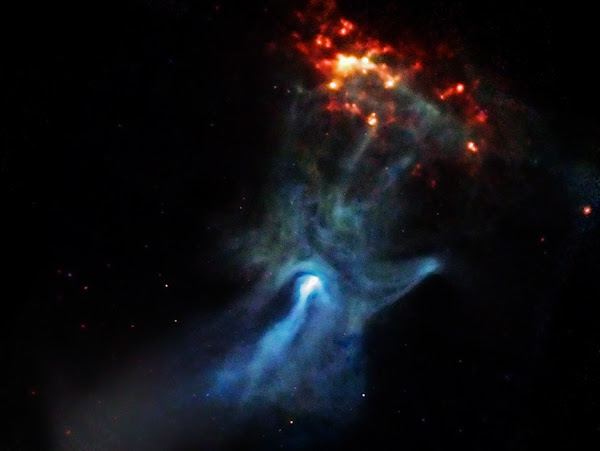### Pulsars to detect Earth's motion

by @ulaulaman about #astronomy #pulsars #mathematics #earth
I published this post some years ago (archived version), but for unilateral decision of the online publisher, it is deleted, so I decide to recover it now.A Pulsar's Hand P. Slane et. al (Apod)

We all known the story of the little green men: in 1967 Jocelyn Bell and Antony Hewish discovered a strange, regular cosmic signal, a periodic bep. They consulted Fred Hoyle, astronomers and sci-fi writer, and he understood that the signal was emitted by a neutrons' star, a pulsar. An italian research team, composed by Matteo Luca Ruggiero, Emiliano Capolongo, Angelo Tartaglia, published the following paper, Pulsars as celestial beacons to detect the motion of the Earth (arXiv). In their paper, researchers propose to apply some relativistic mathematical tools to calculate Earth position using pulsars signals.
First of all, for every pulsars we must define the following 4-vector: $f^\mu = \frac{1}{cT} (1, \hat n)$ where $\hat n$ is the versor of the direction of propagation of pulsar signals.
To each spacetime events we can define the following 4-vector: $r^\mu = (ct, \vec x)$ and using $f$ and $r$, we can construct the following scalar function: $X(r) = f^\mu r_\mu = f \cdot r$ Now, if we have $N$ pulsars, we can construct $N$ scalar functions: $X_{(N)}(r) = f^\mu_{(N)} r_\mu = f \cdot r$ So, starting from the following symmetric matrix $\eta_{(M)(N)} = f_{(M)} \cdot f_{(N)}$ it could be possible calculate an object's position using pulsars' 4-vectors: $r = \sum_a X_{(a)} f^{(a)}$ where $X_{(a)}$ is a phase who corresponds to the coordinates in null frame.
The only problem is now the measure of these phases.
Researchers proposed a metod (I don't try to describe you) that they numerically tested. The most important thing is that reasearchers show the possibility to use pulsars to detect the position of a celestial body.
Ruggiero M.L., Capolongo E. & Tartaglia A. (2011). Pulsars as celestial beacons to detect the motion of the Earth, International Journal of Modern Physics D, 20 (06) 1025-1038. DOI: